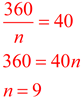Angles and Polygons MathBitsNotebook.com Terms of Use   Contact Person: Donna RobertsInterior Angles

The interior angles of a polygon are the angles at each vertex on the inside of the polygon.
In convex polygons, each interior angle is always less than 180º

Some interior angles are well known and/or easy to find.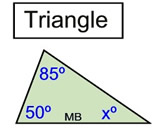We have proven that the sum of the measures of the interior angles of a triangle is 180º. We can easily find that xº = 45º.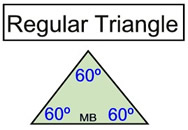A regular triangle is an equilateral triangle with all sides and angles of equal measure. We only need to divide 180 by 3 to find the size of each angle.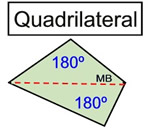When dealing with 4-sided polygons, a diagonal divides the figure into two triangles showing that the sum of the interior angles must be 2(180) = 360º.

The strategy shown in the quadrilateral, of dividing the figure into triangles, will be used to investigate the interior angles of polygons with more than four sides.
This strategy may be referred to as "partitioning" or "dissecting".

If we use diagonals to partition a polygon into a series of triangles (as was done with the quadrilateral), we can calculate the sum of the interior angles of larger-sided polygons.
 In a regular hexagon, four triangles can be created using diagonals of the hexagon from a common vertex. Since the interior angles of each triangle total 180º, the hexagon's interior angles will total 4(180º), or 720º.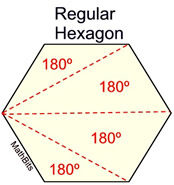This same approach can be taken in an irregular hexagon. The diagonals form four triangles whose interior angles total 180º, giving the hexagon's interior angles a total of 4(180º), or 720º.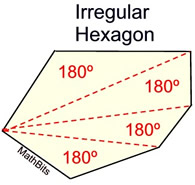Notice that the sum of the interior angles is the same for both the regular and the irregular hexagons. Also notice that we formed 4 triangles in the hexagon, which is 2 less than the number of sides of the polygon. The sum of the interior angles is 180º • (the number of triangles formed) or 180•(n - 2).

The pattern developed in the example above, is consistent for ALL polygons.

 Sum of Interior Angles of a Polygon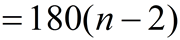(where n = number of sides)

If you know that the polygon is a regular polygon,
you can find each interior angle by dividing by the number of sides.
(Remember that the interior angles of a regular polygon are congruent.)

 Each Interior Angle of a Regular Polygon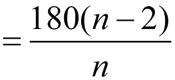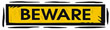You can NOT use this formula to find each angle in an irregular polygon. Since each angle of an irregular polygon may be of different size, there is no formula for finding individual angle measures.When working with angle formulas for polygons, be sure to read carefully to determine what you are being asked to find. Look for "hint" words such as sum, interior, exterior, each, and degrees.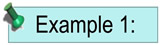Find the number of degrees in the sum of the interior angles of a decagon. A decagon has 10 sides. 180(n - 2) = 180(10 - 2) = 180(8) = 1440º.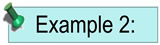How many sides does a polygon have if the sum of the interior angles is 1080º? Set the formula equal to 1080 and solve for n. 180(n - 2) = 1080 180n - 360 = 1080 180n = 1440 n = 8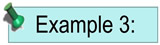Find the number of degrees in each interior angle of a regular pentagon. This is a regular polygon, so we can use the formula. A pentagon has 5 sides.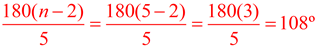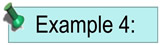Each interior angle of a regular polygon measures 140º. Find the number of sides of the polygon. This is a regular polygon, so use the formula. Set the formula equal to 140 and solve for n.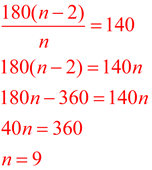Exterior Angles

We discussed the concept of an exterior angle of a triangle in a previous section.
 The exterior angle of a triangle is formed by one side of the triangle and the extension of an adjacent side of the triangle. We will now extend this concept to polygons.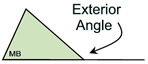The exterior angle of a polygon is an angle that forms a linear pair with one of the interior angles of the polygon. In the same manner as an exterior angle of a triangle, the exterior angle of a polygon is formed by any side of the polygon and the extension of its adjacent side.

 The exterior angle of a polygon is formed by any side of the polygon and the extension of its adjacent side. Exterior angles and interior angles are supplementary and form a linear pair. If the polygon is regular, all of its exterior angles will be the same measure.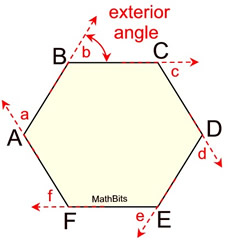There are actually two congruent exterior angles at each vertex, but only ONE will be considered for our work. Shown below, ∠1 and ∠2 are exterior angles and are also congruent vertical angles. Note that ∠3 is NOT an exterior angle, but it is congruent to interior ∠CDE as they are vertical angles.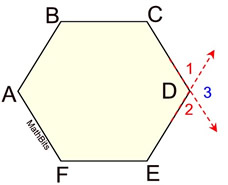If you add ALL exterior angles (ONE at a vertex), you will get a sum of 360º.
The sum of the exterior angles of ALL polygons is a
constant 360º.

 Sum of Exterior Angles of a Polygon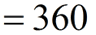If you know that the polygon is a regular polygon,
you can find each exterior angle by dividing by the number of sides, n.

(Remember that the exterior angles of a regular polygon are congruent.)

 Each Exterior Angle of a Regular Polygon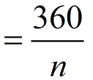So WHY do the exterior angles (taken one at a vertex) always add up to 360º regardless of the number of sides of the polygon?

Here is what we know about exterior angles and polygons:
1. A polygon with n sides will have n interior angles and n exterior angles (one at each vertex).
2. By its formation, an exterior angle is supplementary to its adjacent interior angle.
3. At each vertex of the polygon, the interior angle and the exterior angle form a linear pair. Since there are n vertices, there will be n linear pairs in total around the polygon. Each linear pair adds to 180º for a total of n • 180º or 180n degrees around the polygon.
4. We have already shown that the formula for the sum of the interior angles of a polygon with n sides is 180(n - 2).
5. From the sum of ALL linear pairs (180n), subtract the sum of the interior angles (the formula). You will be left with the sum of the exterior angles.

180n - 180(n - 2) = 180n - 180n + 360 = 0 + 360 = 360.
The sum of the exterior angles is constant (360º) for ALL polygons!!!Find the sum of the exterior angles of a hexagon. Of a pentagon. Of a dodecagon. Of a quadrilateral. Of a 17-gon. All of these answers are the same. The sum of the exterior angles is 360º.How many sides does a polygon have if the sum of the exterior angles is 360º? This is a trick question. The sum of the exterior angles of ALL polygons is 360º. There is insufficient information to determine the number of sides.Find the measure of each exterior angle of a regular octagon. This is a regular polygon, so we can use the formula. An octagon has 8 sides.     360/n = 360/8 = 45ºEach exterior angle of a regular polygon contains 40º. Find the number of sides of the polygon. This is a regular polygon, so use the formula. Set the formula equal to 40 and solve for n.